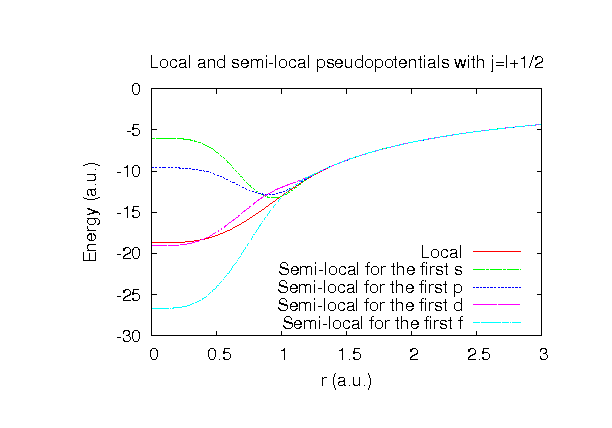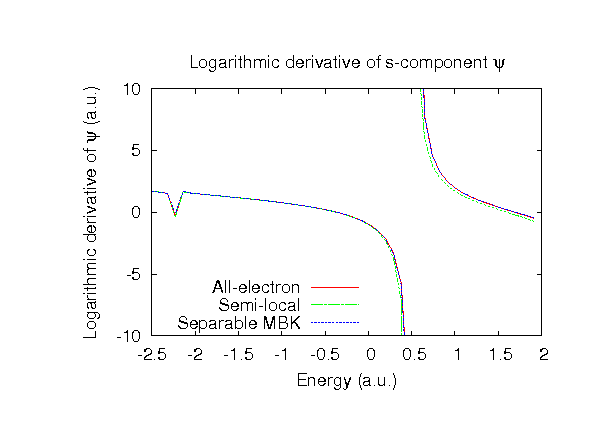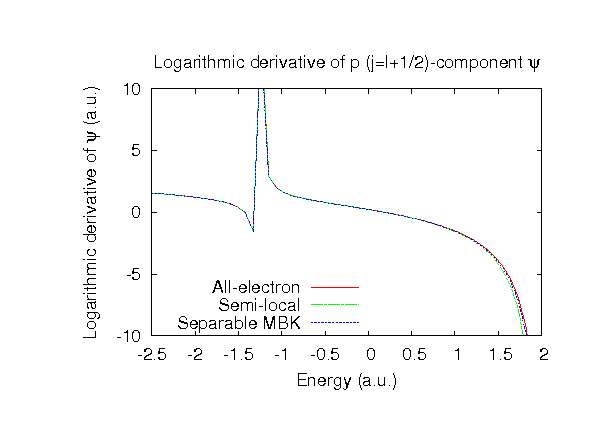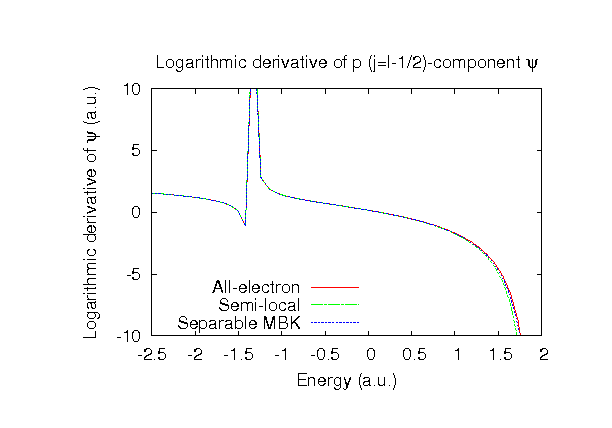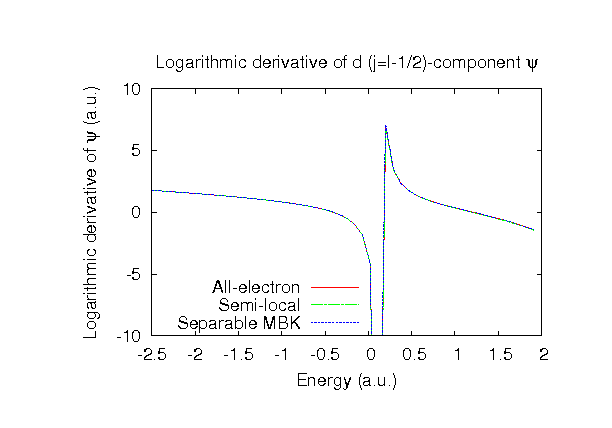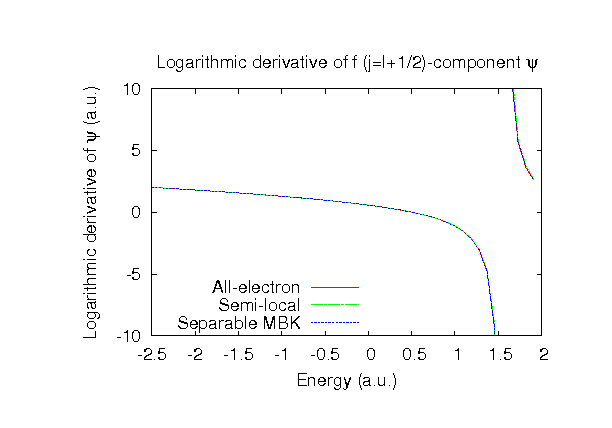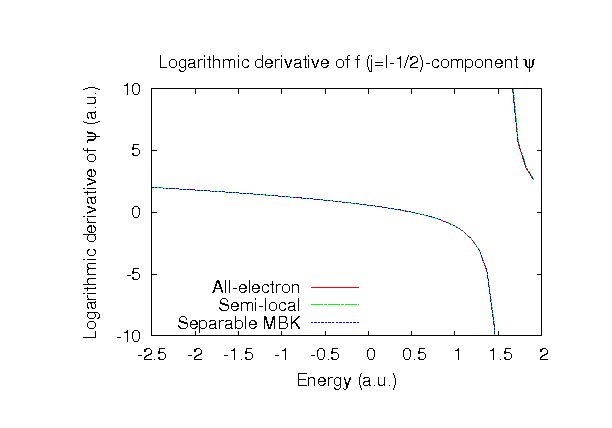### Fully relativistic pseudopotentials

Fully relativistic pseudopotentials generated by the MBK (PRB 47, 6728 (1993)) scheme within LDA (CA13) and GGA (PBE13) which contain a partial core correction and fully relativistic effects including spin-orbit coupling.

### Pseudo-atomic orbitals

The number below the symbol means a cutoff radius (a.u.) of the confinement potential. These file includes fifteen radial parts for each angular momentum quantum number l (=0,1,2,3). The basis functions were generated by variationally optimizing the corresponding primitive basis functions in the single atom, the dimer, and the BCC bulk. The input files used for the orbital optimization can be found at Nb_opt.dat , Nb2_opt.dat , and Nbbcc_opt.dat . Since Nb_CA13.vps and Nb_PBE13.vps include the 4s, 4p, 4d, and 5s states (13 electrons) as the valence states, the minimal basis set is Nb*.*-s2p1d1. Our recommendation for the choice of cutoff radius of basis functions is that Nb7.0.pao is enough for bulks, but Nb9.0.pao or Nb11.0.pao is preferable for molecular systems.

### Benchmark calculations by the PBE13 pseudopotential with the various basis functions

(1) Calculation of the total energy as a function of lattice constant in the bcc structure, where the total energy is plotted relative to the minimum energy for each case. a0 and B0 are the equilibrium lattice constant and bulk modulus obtained by fitting to the Murnaghan equation of state. The difference between Nb7.0-s3p2d2 and Nb7.0-s3p3d3f1 in the total energy at the minimum point is 0.098 eV/atom. An input file used for the OpenMX calculations can be found at Nbbcc-EvsV.dat . For comparison the result by the Wien2k code is also shown, where the calculation was performed by default setting in the Ver. 10.1 of Wien2k except for the use of RMT x KMAX of 12.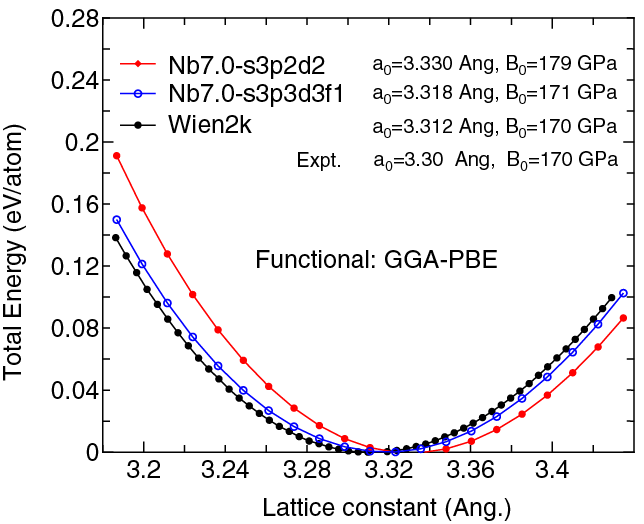(2) Calculations of the band dispersion in the bcc structure, where the non-spin polarized collinear calculation with the lattice constant of 3.30 Ang. was performed using Nb_PBE13.vps, Nb7.0-s3p2d2, and Nb7.0-s3p3d3f1, and the origin of the energy is taken to be the Fermi level. The input file used for the OpenMX calculations can be found at Nbbcc-Band.dat . For comparison the result by the Wien2k code is also shown, where the calculation was performed by default setting in the Ver. 10.1 of Wien2k except for the use of RMT x KMAX of 12.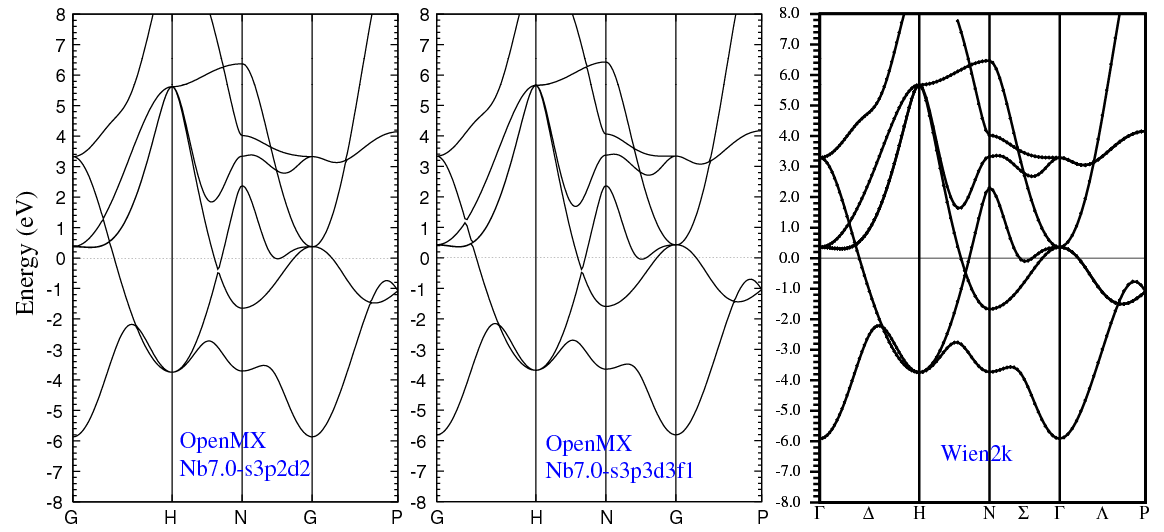(3) Calculations of a niobium molecule with S=3, where Nb_PBE13.vps and Nb9.0-s3p2d2 or Nb9.0-s3p3d3f1 were used. The input files used for the OpenMX calculations can be found at Nb2.dat , Nb.dat , and Nb_cp.dat .

 Equilibrium bond length (Ang.) Atomization energy (eV) Atomization energy (couterpoise corrected) (eV) Nb9.0-s3p2d2 2.164 4.16 4.15 Nb9.0-s3p3d3f1 2.120 4.48 4.45 Other calc. 2.099a 3.912 a Expt. 2.08 b 5.48 b

a B3LYP result: Z.J. Wu, Chem. Phys. Lett. 383, 251 (2004).
b J.R. Lombardi, B. Davis, Chem. Rev. 102, 2431 (2002).

### Supplementary information for the GGA (PBE13) pseudopotential